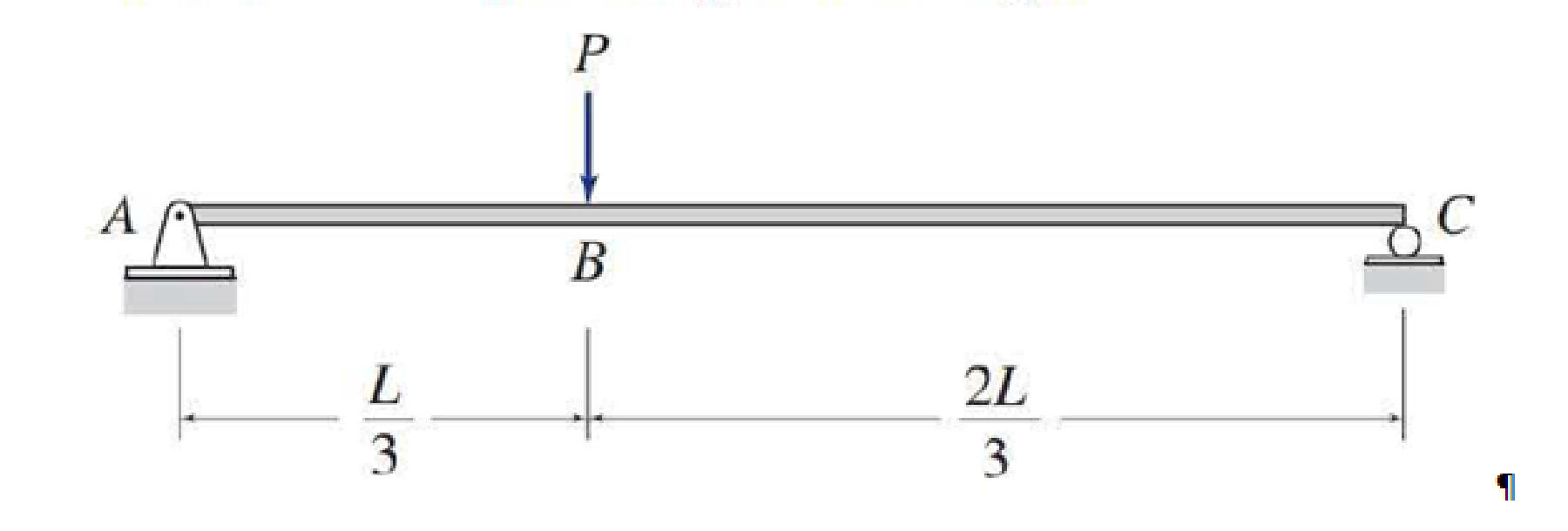# Determine the equations for shear and bending moment for the beam shown. Use the resulting equations to draw the shear and bending moment diagrams.

#### Solutions

Chapter
Section
Chapter 5, Problem 13P
Textbook Problem
4 views

## Determine the equations for shear and bending moment for the beam shown. Use the resulting equations to draw the shear and bending moment diagrams.To determine

Find the equations for the shear and bending moment of the beam.

Plot the shear and bending moment diagram.

### Explanation of Solution

Sign conversion:

Apply the sign convention for calculating the equations of equilibrium as below.

• For the horizontal forces equilibrium condition, take the force acting towards right side as positive (+) and the force acting towards left side as negative ().
• For the vertical forces equilibrium condition, take the upward force as positive (+) and downward force as negative ().
• For moment equilibrium condition, take the clockwise moment as negative and counter clockwise moment as positive.

Apply the following sign convention for calculating the shear and bending moments.

• When the portion of the beam considered is left of the section, then the external force acting to the left are considered as positive.
• When the portion of the beam considered is right of the section, then the external force acting to the right are considered as positive.
• When the portion of the beam considered is left of the section, then the external force acting upward are considered as positive.
• When the portion of the beam considered is right of the section, then the external force acting downward are considered as positive.
• When the portion of the beam considered is left of the section, then the clockwise moments are considered as positive.
• When the portion of the beam considered is right of the section, then the counterclockwise moments are considered as positive.

Calculation:

Show the free-body diagram of the entire beam as in Figure 1.

Find the horizontal reaction at point A by resolving the horizontal equilibrium.

+Fx=0Ax=0Ax=0

Find the vertical reaction at point C by taking moment about point A.

+MA=0P(L3)+Cy(L)=0Cy=P3

Find the vertical reaction at point A by resolving the vertical equilibrium.

+Fy=0AyP+Cy=0AyP+P3=0Ay=2P3

Pass the section aa and bb at a distance x from left end of the beam.

Show the section aa and bb in the entire beam as in Figure 2.

Consider section AB:

Consider the left side of the section aa for calculation of shear and bending moment.

Show the free-body diagram of the left side of the section aa as in Figure 3.

Find the shear at section aa by resolving the vertical equilibrium.

+Fy=02P3S=0S=2P3for0<x<L3

Find the moment at section aa by taking moment about the section aa.

+M=02P3(x)M=0M=2Px3for0<x<L3

Consider section BC:

Consider the left side of the section bb for calculation of shear and bending moment

### Still sussing out bartleby?

Check out a sample textbook solution.

See a sample solution

#### The Solution to Your Study Problems

Bartleby provides explanations to thousands of textbook problems written by our experts, many with advanced degrees!

Get Started

Find more solutions based on key concepts
What is the relationship between mass flow rate and volume flow rate?

Engineering Fundamentals: An Introduction to Engineering (MindTap Course List)

What should be done as a drill nears the breakthrough point during drilling?

Precision Machining Technology (MindTap Course List)

Identify the six basic components of any computer-based information system.

Principles of Information Systems (MindTap Course List)

List characteristics of a smartphone.

Enhanced Discovering Computers 2017 (Shelly Cashman Series) (MindTap Course List)

The homogeneous 340-lb sign is suspended from three wires. Find the tension in each wire.

International Edition---engineering Mechanics: Statics, 4th Edition

If your motherboard supports ECC DDR3 memory, can you substitute non-ECC DDR3 memory?

A+ Guide to Hardware (Standalone Book) (MindTap Course List)